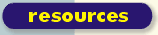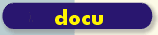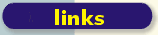scsh.net comp.lang.scheme.scsh 2003 ```Date: Wed, 19 Feb 2003 01:15:42 -0000 From: Tom Lord Subject: Re: Usage of Shivers' SRE regular expression notation Alex Shin: Most regular expression operations I find are concatenations of other regular expressions, so this works out well. You can also define higher order regular expression functions, with only slightly less convenience than in an sexp syntax, but that's of questionable use to begin with. If you need that much structure, regular expressions are probably the wrong tool. Something that bridges the gap between regular expressions and full grammars (i.e. what Perl 6 plans) would be great in Scheme, but that doesn't seem to be what SRE's are for. Generally, I'm a huge advocate of sexp's over other syntax in almost every case. But the above example looks about as simple as you can hope for in Perl. The SRE version has about the same number of conceptual elements, but is much more verbose and "looks" nothing like what you're trying to match. I'd like to see an example where SRE really shines over conventional regexp syntax. Both the forum and the status of most regexp engine implementations fight against a good response. Both demand a fairly small reply, yet one of the most significant aspects of SRE's is that they scale nicely to quite large examples. I use a close variant of Olin's SRE syntax, backed by my own regexp engine. Enclosed is a page from my Scheme-based wiki -- I'm not confident it'll give you a clear picture -- but at least it should give you a hint. Let me call your attention to some aspects of this code. First, a _huge_ regexp is expressed in lots of separate clauses, each of which uses SRE syntax. Application code which processes this definition composes the separate clauses into a massive SRE using simple list operators (map and backquote). That code doesn't have to fuss with generating strings that happen to satisfy regcomp -- the list structure nicely reflects the intended structure of the regexp. It's a very natural-feeling way to program. Second, SRE-implementation code which processes the resulting massive SRE uses simple list operations to, for example, identify keywords with matching clauses of a regexp "|" expression. In short, SRE makes manipulating regexps feel to a Scheme programmer just as natural (and quite similar) to manipulating Scheme source code in s-exp form. I can sling around SRE fragments quite robustly using just map and backquote, without having to think too hard about the peculiarities of `regcomps' surface syntax. In contrast, Perl's structured regexp syntax requires regexp-specific string mungers to work with similarly. Quite honestly, when I first saw Perl's introduction of a "structured" regexp syntax, I thought, "hey, that's funny: it's an SRE parody." Finally, let me add that a little bit of Emacs bigotry. I navigate and edit my SREs using emacs s-exp navigation and edit commands. Some people never get past the set of operators present in, say, vi -- but those of us who have that capability -- well, we appreciate an s-exp notation for the precision and ease with which it can be manipulated by an elegant editor such as Emacs. Another way to say the same thing: what's wrong with you that you don't find obvious value in an economy of notational concepts? -t ;;;;;;;;;;;;;;;;;;;;;;;;;;;;;;;;;;;;;;;;;;;;;;;;;;;;;;;;;;;;;;;; ;;; Division of Input into Typed Paragraphs ;;; (begin (define wiki-paragraph-rules ;; (type test separator) ;; ;; Test is a structured regexp to be compiled in a larg `(| ...)' ;; of all of the test expressions. The leftmost-longest matching ;; test expression determines the type of the first paragraph in a ;; given string. ;; ;; `(separator string)' returns a list: `(paragraph remaining-string)', ;; separating the first paragraph from the rest of the string. ;; ;; The `test' expression and `separator' procedure can safely assume ;; that the string is not empty, and does not begin with any blank lines. ;; `((:form-feed (& (* ([] blank)) "\f" (* ([] blank))) ,(lambda (s) (one-line-separator s))) (:comment-line (& "%%%"(* ([^] "\n"))) ,(lambda (s) (one-line-separator s))) (:rfc822ish (& (* ([] blank)) "+++" (* ([] blank))) ,(lambda (s) (ordinary-paragraph-separator s))) (:title (& "!" (+ ([^] "\n"))) ,(lambda (s) (ordinary-paragraph-separator s))) (:card-boundary "\f---" ,(lambda (s) (one-line-separator s))) (:heading (& (* ([] blank)) (+ "*") ([] blank) ([^] ")#*\n") (* ([^] "\n"))) ,(lambda (s) (ordinary-paragraph-separator s))) (:menu (& (* ([] blank)) "-*-*-" (* ([] blank))) ,(lambda (s) (one-line-separator s))) (:verbatim (& (* ([] blank)) "<<<" (* ([] blank))) ,(lambda (s) (verbatim-paragraph-separator s))) (:small-paragraph (& (* ([] blank)) "(((" (* ([] blank))) ,(lambda (s) (small-paragraph-separator s))) (:text-area (& (* ([] blank)) "?<<<" (* ([^] #\nl))) ,(lambda (s) (verbatim-paragraph-separator s))) (:one-line-verbatim (& "#" (* ([^] "\n"))) ,(lambda (s) (one-line-separator s))) (:separator-line (& (* ([] blank)) "---" (* "-") (* ([] blank))) ,(lambda (s) (one-line-separator s))) (:horizontal-rule (& (* ([] blank)) "===" (* "=") (* ([] blank))) ,(lambda (s) (one-line-separator s))) (:poetry-start (& (* ([] blank)) "\\\\" (* ([] blank)) (? "//") (* ([] blank))) ,(lambda (s) (one-line-separator s))) (:i-poetry-start (& (* ([] blank)) "\\\\_" (* ([] blank)) (? (* "_") "//") (* ([] blank))) ,(lambda (s) (one-line-separator s))) (:ib-poetry-start (& (* ([] blank)) "\\\\__" (* ([] blank)) (? (* "_") "//") (* ([] blank))) ,(lambda (s) (one-line-separator s))) (:poetry-end (& (* ([] blank)) "//" (* ([] blank)) (? "\\\\") (* ([] blank))) ,(lambda (s) (one-line-separator s))) (:bullet-item (& (* ([] blank)) (+ "*") ")" (* ([^] "\n"))) ,(lambda (s) (ordinary-paragraph-separator s))) (:definitions-item (& (* ([] blank)) "+" (* ([^] "\n")) (| (& ":::" (* ([^] "\n"))) (& "+" (* ([] blank))))) ,(lambda (s) (ordinary-paragraph-separator s))) (:numbered-item (& (* ([] blank)) (+ "*") "#" (* ([^] "\n"))) ,(lambda (s) (ordinary-paragraph-separator s))) (:poetry-display (& (* ([] blank)) "~~~" (* "~") (* ([] blank))) ,(lambda (s) (one-line-separator s))) (:display-separator (& (* ([] blank)) "###" (* "#") (* ([] blank))) ,(lambda (s) (one-line-separator s))) (:begin-block-quote (& (* ([] blank)) "```" (* "`") (* ([] blank))) ,(lambda (s) (one-line-separator s))) (:end-block-quote (& (* ([] blank)) "'''" (* "'") (* ([] blank))) ,(lambda (s) (one-line-separator s))) (:table-start (& (* ([] blank)) (+ "v") "===" (* ([^] "\n")) (* "=") (* "v") (* ([] blank))) ,(lambda (s) (one-line-separator s))) (:table-end (& (* ([] blank)) (+ "^") "===" (* "=") (* "^") (* ([] blank))) ,(lambda (s) (one-line-separator s))) (:meta-x-form (& (* ([] blank)) "?M-x" (+ ([] blank)) (+ ([] alnum "-")) (* ([] blank))) ,(lambda (s) (one-line-separator s))) (:form-start (& (* ([] blank)) (+ "v") "???" (* "?") (* ([^] "\n"))) ,(lambda (s) (one-line-separator s))) (:form-end (& (* ([] blank)) (+ "^") "???" (* "?") (* ([] blank))) ,(lambda (s) (one-line-separator s))) (:section-name (& (* ([] blank)) "::" (* ([^] "\n")) "::" (* ([] blank))) ,(lambda (s) (one-line-separator s))) (:note-name (& (* ([] blank)) "{" (* ([^] "}")) "}::" (* ([] blank))) ,(lambda (s) (one-line-separator s))) (:biblio-name (& (* ([] blank)) "[" (* ([^] "]")) "]::" (* ([^] "\n"))) ,(lambda (s) (one-line-separator s))) (:include-topic (& (* ([] blank)) "@@" (* ([^] "\n"))) ,(lambda (s) (one-line-separator s))) (:short-table (& (* ([] blank)) "|" (* ([^] "\n")) "|") ,(lambda (s) (one-line-separator s))) )) (define identify-paragraph-type (let ((regexp (structured-regexp->procedure `(^\$ (| ,@(map (lambda (rule) `(! ,(car rule) ,(cadr rule))) wiki-paragraph-rules))) :pick-spec '?))) (lambda (s) (if (string-null? s) :eof (let ((re-case (regexp (make-shared-substring s 0 (or (string-index s #\nl) (string-length s)))))) (or re-case :regular-paragraph)))))) (define ordinary-paragraph-separator (let ((pattern (structured-regexp->procedure `(^\$ (| (* ([] blank)) ,@(map (lambda (rule) `,(cadr rule)) wiki-paragraph-rules))) :cflags 'REG_NEWLINE :eflags 'REG_NOTBOL :pick-spec '(< (0 >))))) (lambda (s) (or (pattern s) (list s ""))))) (define verbatim-paragraph-separator (let ((pattern (structured-regexp->procedure `(^ ">>>\n") :cflags 'REG_NEWLINE :pick-spec '((< 0) >)))) (lambda (s) (or (pattern s) (list (string-append s "\n>>>\n" "")))))) (define small-paragraph-separator (let ((pattern (structured-regexp->procedure `(^ ")))\n") :cflags 'REG_NEWLINE :pick-spec '((< 0) >)))) (lambda (s) (or (pattern s) (list (string-append s "\n)))\n" "")))))) (define one-line-separator (structured-regexp->procedure `(& (* ([^] "\n")) (| "\n" (\$ ""))) :pick-spec '(0 >)))) ;; (wiki-paragraph-lexer string) ;; ;; Return a procedure `lexer'. ;; ;; Calling lexer with no arguents return successive paragraphs from `string' ;; in the form: ;; ;; (type paragraph) ;; ;; where `type' is one of the keywords from the table `wiki-paragraph-rules' ;; and `paragraph' a string. ;; ;; Passing a single true argument causes the same return value, but the ;; paragraph is not removed from the stream. ;; (define (wiki-paragraph-lexer initial-string) (let* ((known-next-lexeme #f) (string "") (lexer-procedure (lambda (:optional peek?) (if (not known-next-lexeme) (set! known-next-lexeme (wiki-lex-paragraph (without-initial-blank-lines string)))) (let ((answer (list (car known-next-lexeme) (cadr known-next-lexeme)))) (if (not peek?) (begin (set! string (caddr known-next-lexeme)) (set! known-next-lexeme #f))) answer)))) (set! string initial-string) lexer-procedure)) (define without-initial-blank-lines (let ((ordinary-case (structured-regexp->procedure `(^ (* (& (* ([] blank)) "\n"))) :pick-spec '>)) (all-blanks? (structured-regexp->procedure `(^\$ (* ([] blank)))))) (lambda (s) (cond ((string-index s #\nl) (ordinary-case s)) ((all-blanks? s) "") (#t s))))) (define (wiki-lex-paragraph string) (let* ((type (identify-paragraph-type string)) (rule (or (assq type wiki-paragraph-rules) (if (string-null? string) `(:eof #f ,(lambda (s) (list "" ""))) `(:regular-paragraph #f ,(lambda (s) (ordinary-paragraph-separator s))))))) (cons type ((caddr rule) string)))) ``` ```Date: Thu, 20 Feb 2003 11:29:30 +0900 From: Alex Shinn Subject: Re: Usage of Shivers' SRE regular expression notation Tom> I use a close variant of Olin's SRE syntax, backed by my own Tom> regexp engine. Enclosed is a page from my Scheme-based wiki -- Tom> I'm not confident it'll give you a clear picture -- but at Tom> least it should give you a hint. This is a very nice example of where regular expressions are a good solution. I've never seen a Wiki written with a non-regexp parser. It also factors very well, so you could emphasize the sexp advantage if you wrote it like: ;; common regexp patterns (define opt-ws '(* ([] blank))) (define in-line '(* ([^] "\n"))) (define (in-ws . args) (append `(& ,opt-ws) args `(,opt-ws))) (define (start-line . args) (append `(& ,opt-ws) args `(,in-line))) (define wiki-paragraph-rules (map ;; default separator to one-line-separator (lambda (x) (if (pair? (cddr x)) x (append x (list one-line-separator)))) `((:form-feed ,(in-ws "\f")) (:comment-line (& "%%%"(* ([^] "\n")))) (:rfc822ish ,(in-ws "+++") ,ordinary-paragraph-separator) (:title (& "!" (+ ([^] "\n"))) ,ordinary-paragraph-separator) (:card-boundary "\f---") (:heading (& ,opt-ws (+ "*") ([] blank) ([^] ")#*\n") ,in-line) ,ordinary-paragraph-separator) (:menu ,(in-ws "-*-*-")) (:verbatim ,(in-ws "<<<") ,verbatim-paragraph-separator) (:small-paragraph ,(in-ws "(((") ,small-paragraph-separator) (:text-area ,(in-ws "?<<<") ,verbatim-paragraph-separator) (:one-line-verbatim (& "#" (* ([^] "\n")))) (:separator-line ,(in-ws "---" '(* "-"))) (:horizontal-rule ,(in-ws "===" '(* "="))) (:poetry-start ,(in-ws "\\\\" opt-ws '(? "//"))) (:i-poetry-start ,(in-ws "\\\\_" opt-ws '(? (* "_") "//"))) (:ib-poetry-start ,(in-ws "\\\\__" opt-ws '(? (* "_") "//"))) (:poetry-end ,(in-ws "//" opt-ws '(? "\\\\"))) (:bullet-item ,(start-line '(+ "*") ")") ,ordinary-paragraph-separator) (:definitions-item (& ,opt-ws "+" ,in-line (| (& ":::" ,in-line) (& "+" ,opt-ws))) ,ordinary-paragraph-separator) (:numbered-item ,(start-line '(+ "*") "#") ,ordinary-paragraph-separator) (:poetry-display ,(in-ws "~~~" '(* "~"))) (:display-separator ,(in-ws "###" '(* "#"))) (:begin-block-quote ,(in-ws "```" '(* "`"))) (:end-block-quote ,(in-ws "'''" '(* "'"))) (:table-start ,(in-ws '(+ "v") "===" in-line '(* "=") '(* "v"))) (:table-end ,(in-ws '(+ "^") "===" '(* "=") '(* "^"))) (:meta-x-form ,(in-ws "?M-x" opt-ws '(+ ([] alnum "-")))) (:form-start ,(start-line '(+ "v") "???" '(* "?"))) (:form-end ,(in-ws '(+ "^") "???" '(* "?"))) (:section-name ,(in-ws "::" in-line "::")) (:note-name ,(in-ws "{" '(* ([^] "}")) "}::")) (:biblio-name ,(start-line "[" '(* ([^] "]")) "]::")) (:include-topic ,(start-line "@@")) (:short-table (& ,opt-ws "|" ,in-line "|")) ))) However, this style of regexp building is reminiscent of the way Emacs builds dynamic fontification regexps. When constructing regexps from smaller regexps, an sexp offers no advantage over a string. The advantage of an sexp is that it retains its structure, so you can provide introspection and mapping over the individual parts of the regexp. As an example, suppose your regexp engine didn't support case-insensitivity. With an sexp notation, you could easily write a procedure that traverses the regexp, converting strings to sequences of optional upper/lowercase characters. With a large amount of effort you could accomplish the same the with a string regexp (it would mean parsing the string), and more complex examples become increasingly unwieldy. That's the canonical example of the power of sexps - adding a new intrinsic feature (like objects, or case-insensitivity) where no such feature existed before. In this case, once you have an SRE parser it's easy to write procedures to/from that notation and strings. Which notation you use is then more a matter of style; most regexps will be shorter as a string, but you do have the awkwardness of the extra \\ quoting that was mentioned. A more interesting question is therefore whether the core language should support regexps with a // style syntax. This allows even more concise notation, avoids the \\ confusion, and can be computed at compile-time. The Scheme way is not to add any non-essential features to the language, but if you do heavy regexp processing this is worthwhile. In much the same way, if you write a lot of mathematical formulas you will invariably want an infix notation (insert flamewar here). In these cases it might be nice to add support for syntax extensions to the language, so things like regular expression syntax can be done in a library. SRFI-10 doesn't count because it still requires everything be an sexp. Guile, for instance, would let you define a #// style regexp syntax that converts to an SRE. Tom> Finally, let me add that a little bit of Emacs bigotry. I Tom> navigate and edit my SREs using emacs s-exp navigation and edit Tom> commands. Emacs navigates group nesting in string regexps just fine. -- Alex ``` ```Date: Thu, 20 Feb 2003 06:44:19 -0000 From: Tom Lord Subject: Re: Usage of Shivers' SRE regular expression notation Tom> Finally, let me add that a little bit of Emacs bigotry. I Tom> navigate and edit my SREs using emacs s-exp navigation and edit Tom> commands. Emacs navigates group nesting in string regexps just fine. No it can't. (Consider regexps containing character sets like "[]abc]".) A lot of what we agree about could be summed up as "economy of concepts and mechanisms". Given the idea of an s-exp, and a mechanism for manipulating them, it just plain "works out nicely" to use that idea and those mechanisms for every problem they're isomorphic too. But contrary to that, another point we both agree about, is that infix, punctuation-heavy, domain-specific syntaxes have their place. -t ``` ```Date: Thu, 20 Feb 2003 09:28:24 +0100 From: Michel Schinz Subject: Re: Usage of Shivers' SRE regular expression notation Alex Shinn writes: [...] > However, this style of regexp building is reminiscent of the way > Emacs builds dynamic fontification regexps. When constructing > regexps from smaller regexps, an sexp offers no advantage over a > string. Well, the regexps used in Emacs for fontification do provide, in my opinion, a very good argument for sexps. At least if you temporarily go back to the days of Emacs 20. Emacs provides regexp-opt which is used to create optimised regexps matching a set of keywords. For example, if you give it the keywords "if", "import" and "in" it outputs something like "i\\(mport\\|[fn]\\)". This is typically used to create regular expressions for syntax highlighting. Now, often you want to build a big regular expression which matches such a set of keywords and, say, the identifier which follows, because you want to highlight that identifier too. The problem is that you do not know how many groups (or submatches, in scsh terminology) were created by regexp-opt, and therefore you do not know the number of the group containing the identifier which follows the keyword. For that reason, Emacs provides regexp-opt-depth which counts the number of groups in a regexp. It can be used to solve, in an ugly way I think, the above problem: you use regexp-opt to create the regular expression matching the set of keywords, then you count the number of groups it contains with regexp-opt-depth, and you use that information to compute the group number of the identifier which follows. The scsh solution to that same problem is a lot better, I think: by default, when you build big regexps out of small ones, submatches are removed. And if you really want to keep them, you can, of course. Now what I said applies to Emacs 20 because with Emacs 21 (IIRC), so-called shy groups were introduced (which also exist in Perl and certainly elsewhere). These shy groups do group parts of the regexp but they do not count as submatches. Regexp-opt now creates only shy groups, and that solves the problem in that particular case, but in that particular case only. However, the general problem remains: when you want to combine several small regexps to create a big one, and you do not know how many groups are contained in the small ones, you have a problem. And this problem is easily solved with SREs because the regexps are represented as an ADT which is a lot easier to manipulate programmatically than a string, as all compiler authors know. Now to be fair all this is not due to the SRE notation itself, but to the fact that scsh internally represents regexps as an ADT, and lets the user access that ADT. One could also write a parser for string-based regexps (i.e. scsh's posix-string->regexp) and do all these manipulations on the ADT, like removing groups or turning them into shy groups, before sending the regexp to the underlying engine, maybe as a string. Michel. ``` Up